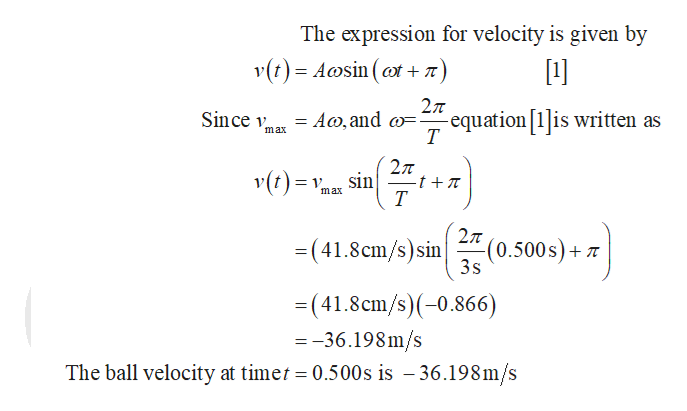# A ball is attached to a spring on a frictionless horizontal surface. The ball is pulled to the right and released from rest at t = 0 s. If the ball reaches a maximum speed of 41.8 cm/s and oscillates with a period of 3.00 s, what is the ball's velocity at t = 0.500 s?

Question
30 views

A ball is attached to a spring on a frictionless horizontal surface. The ball is pulled to the right and released from rest at t = 0 s. If the ball reaches a maximum speed of 41.8 cm/s and oscillates with a period of 3.00 s, what is the ball's velocity at t = 0.500 s?

check_circle

Step 1help_outlineImage TranscriptioncloseThe expression for velocity is given by v(t) Acosin (7) 27T Since v = A0,and o=. -equation is written as Т max 2л sin v() -t + max Т 2л -(0.500s) T 3s =(41.8cm/s)sin (41.8cm/s)(-0.866) =-36.198m/s The ball velocity at timet 0.500s is -36.198m/s fullscreen

### Want to see the full answer?

See Solution

#### Want to see this answer and more?

Solutions are written by subject experts who are available 24/7. Questions are typically answered within 1 hour.*

See Solution
*Response times may vary by subject and question.
Tagged in

### Physics